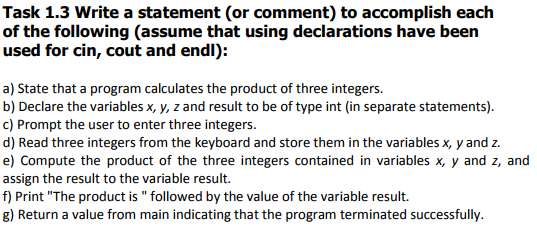# Question & Answer: rite a statement (or comment) to accomplish each of the following (assume that using declarations…..Write a statement (or comment) to accomplish each of the following (assume that using declarations have been used for cin, cout and endl): a) State that a program calculates the product of three integers. b) Declare the variables x, y, z and result to be of type int (in separate statements). c) Prompt the user to enter three integers. d) Read three integers from the keyboard and store them in the variables x, y and z. e) Compute the product of the three integers contained in variables x, y and z, and assign the result to the variable result. f) Print “The product is” followed by the value of the variable result. g) Return a value from main indicating that the program terminated successfully.

Don't use plagiarized sources. Get Your Custom Essay on
Question & Answer: rite a statement (or comment) to accomplish each of the following (assume that using declarations…..
GET AN ESSAY WRITTEN FOR YOU FROM AS LOW AS \$13/PAGE

Assuming C++ the following code is written

a. //This program calculates the product of three integers

In C++ we use // for single line comment or /* comment */ for multiple line comment (replace comment by what ever you want. A comment is a line of code that compiler ignores.

b. int x;

int y;

int z;

To declare variable just write the data type followed by the name of the variable

c. cout<<“Enter three integers: “<<endl;

This will print out a statement saying Enter three integers followed by a new line

d. cin>>x>>y>>z;

This will take 3 inputs irrespective of data type. They can be all same or different data types but consistent with the declaration.

e. int result = x * y * z;

This declares a result variable and assigns it a value of the product of x y and z.

f. cout<<“The product is “<<result;

Similar to the above cout statement but this also takes a variable.

g. return 0;

0 is usually used to show successful termination of program but can use anything